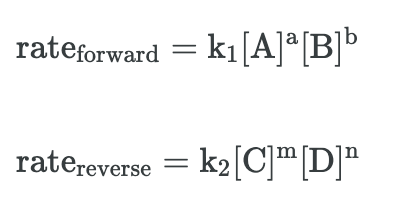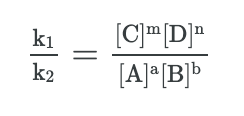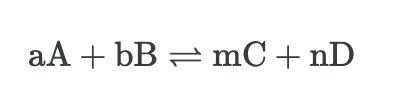Cyber Monday Sale: ALL MCAT Products & Admissions Services

MCAT Content / Rate Processes In Chemical Reactions Kinetics And Equilibrium / Equilibrium In Reversible Chemical Reactions

### Equilibrium in Reversible Chemical Reactions

Topic: Rate Processes In Chemical Reactions Kinetics And Equilibrium

In a chemical equilibrium, the forward and reverse reactions occur at equal rates, and the concentrations of products and reactants remain constant.

Law of Mass Action

The Law of Mass Action links the rate of a chemical reaction as proportional to the concentrations of the reactants and products in a chemical reaction. For a chemical reaction mixture that is in equilibrium, the ratio between the concentration of the reactants and products is constant. This allows for equilibrium constants to be calculated using kinetic data on the rate of a reaction and the rate equation. We can, therefore, raise the stoichiometric coefficients in the rate equation to the power of the concentrations of reactants and products to calculate the equilibrium constant.

The law of mass action can be used to derive the equilibrium constant as when considering the equation:The forward and backward rate can be considered as:However, we know that the forward and reverse reaction rates are equal in equilibrium:Rearranging this equation and separating the rate constants from the concentration terms, we get:Notice that the left side of the equation is the quotient of two constants, which is simply another constant. We simplify and write this constant as Keq:Equilibrium Constant

The equilibrium constant (Keq) is the ratio of the mathematical product of the concentrations of the products of a reaction to the mathematical product of the concentrations of the reactants of the reaction. For example, for a reversible reaction with the equation:This expression is written in terms of the concentrations of the various reactants and products and is given by:Species in brackets represent the concentrations of products, which are always in the numerator, and reactants, which are always in the denominator. Each of the concentrations is raised to a power equal to the stoichiometric coefficient for each species.

Le Chatelier’s Principle Application

Le Chatelier’s principle states that changes to an equilibrium system will result in a predictable shift that will counteract the change. It can be used to predict the behavior of a system due to changes in pressure, temperature, or concentration.

For temperature with the addition of heat to a reaction will favor the endothermic direction of a reaction as this reduces the amount of heat produced in the system.

Increasing the concentration of reactants will drive the reaction to the right while increasing the concentration of products will drive the reaction to the left.

A change in pressure or volume will result in an attempt to restore equilibrium by creating more or fewer moles of gas. For example, if the pressure in a system increases or the volume decreases, the equilibrium will shift to favor the side of the reaction that involves fewer moles of gas. Similarly, if the volume of a system increases or the pressure decreases, the production of additional moles of gas will be favored.

Catalysts do not affect the equilibrium state of a reaction.

Practice Questions

Basic concepts in bioenergetics: phosphoryl group transfers and ATP hydrolysis

Applications of Hard-Soft Acid-Base theory

MCAT Official Prep (AAMC)

Chemistry Online Flashcards Question 20

Official Guide C/P Section Passage 1 Question 3

Chemistry Question Pack Passage 4 Question 21

Chemistry Question Pack Passage 4 Question 22

Chemistry Question Pack Passage 13 Question 74

Practice Exam 1 C/P Section Question 57

Practice Exam 2 C/P Section Passage 6 Question 30

Practice Exam 2 C/P Section Passage 7 Question 37

Practice Exam 3 C/P Section Passage 10 Question 56

Sample Test B/B Section Question 15

Sample Test B/B Section Passage 9 Question 50

Practice Exam 2 B/B Section Passage 2 Question 5

Practice Exam 4 B/B Section Passage 6 Question 33

Key Points

• The law of mass action can be used to derive the equilibrium constant by examing the rate of the forwards and backward reaction in equilibrium and deriving this into an equation that considers the impact of the molar coefficients from the rate equation

• The equilibrium constant can be used to determine the progression of a reaction by measuring the ratios of products to reactants

• Le Chatelier’s principle states that changes to an equilibrium system will result in a predictable shift that will counteract the change. Temperature, pressure, and concentration can all cause equilibrium to shift whereas a catalyst does not affect the position of the equilibrium.

Key Terms

Chemical equilibrium: In a chemical reaction, the state in which both reactants and products are present at concentrations that have no further tendency to change with time.

Equilibrium position: The point in a chemical reaction at which the concentrations of reactants and products are no longer changing.

Law of Mass Action: Links the rate of a chemical reaction as proportional to the concentrations of the reactants and products in a chemical reaction.

Equilibrium constant (Keq): The ratio of the mathematical product of the concentrations of the products of a reaction to the mathematical product of the concentrations of the reactants of the reaction

Le Chatelier’s principle: States that changes to an equilibrium system will result in a predictable shift that will counteract the change

Billing Information